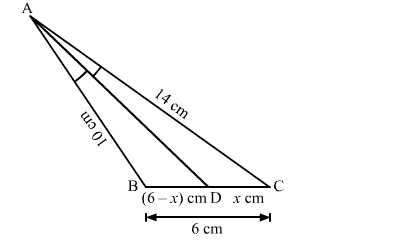# In a △ABC, it is given that AD is the internal bisector of ∠A.Question:

In a △ABC, it is given that AD is the internal bisector of ∠A. If AB = 10 cm, AC = 14 cm and BC = 6 cm, the CD = ?
(a) 4.8 cm
(b) 3.5 cm
(c) 7 cm
(d) 10.5 cmSolution:

By using angle bisector theore in △ABC, we have

$\frac{\mathrm{AB}}{\mathrm{AC}}=\frac{\mathrm{BD}}{\mathrm{DC}}$

$\Rightarrow \frac{10}{14}=\frac{6-x}{x}$

$\Rightarrow 10 x=84-14 x$

$\Rightarrow 24 x=84$

$\Rightarrow x=3.5$

Hence, the correct answer is option (b).